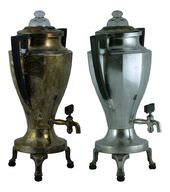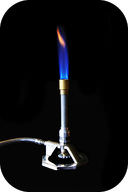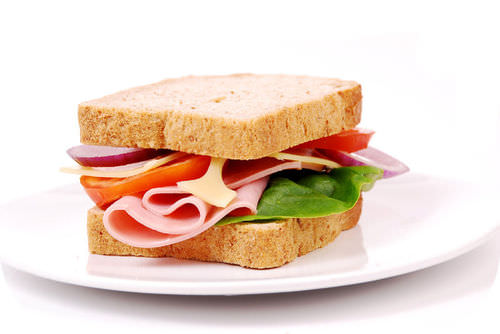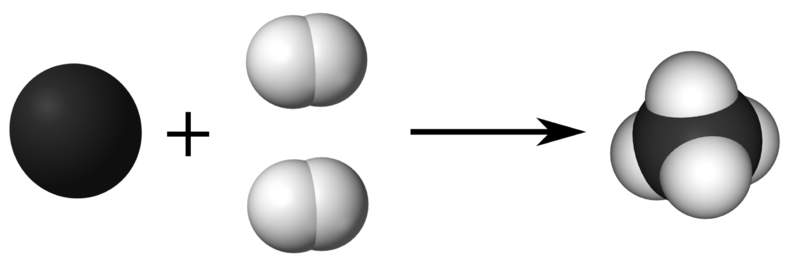# 10.5: Chemical Equations

•• Contributed by Allison Soult
• Senior Lecturer (Chemistry) at University of Kentucky

Learning Outcomes

• Describe chemical reactions using word equations.
• Write equations for chemical reactions.
• Use coefficients to balance chemical equations.

Chemical reactions are occurring all around you. Plants use sunlight to drive their photosynthetic process and produce energy. Cars and other vehicles burn gasoline in order to power their engines. Batteries use electrochemical reactions to produce energy and power many everyday devices. Many chemical reactions are going on inside you as well, especially during the digestion of food.

In math class, you have written and solved many mathematical equations. Chemists keep track of chemical reactions by writing equations as well. In any chemical reaction one or more substances, called reactants, are converted into one or more new substance, called products. The general form of the equation for such a process looks like this.

$\text{Reactants} \rightarrow \text{Products}$

Unlike in a math equation, a chemical equation does not use an equal sign. Instead the arrow is called a yield sign and so the equation is described as "reactants yield products".

### Word Equations

You can describe a chemical reaction by writing a word equation. When silver metal is exposed to sulfur it reacts to form silver sulfide. Silver sulfide is commonly known as tarnish and turns the surface of silver objects dark and streaky black (see figure below). The sulfur that contributes to tarnish can come from traces of sulfur in the air or from food such as eggs. The word equation for the process is:

$\text{Silver} + \text{sulfur} \rightarrow \text{Silver sulfide}$

The silver and the sulfur are the reactants in the equation, while the silver sulfide is the product.Figure $$\PageIndex{1}$$: The coffee percolator on the left has been tarnished from exposure to sulfur. Tarnish is the chemical compound silver sulfide. The same percolator on the right has been polished with a tarnish removal product in order to restore its silver finish.

Another common chemical reaction is the burning of methane gas. Methane is the major component of natural gas and is commonly burned on a gas stove or in a Bunsen burner (see figure below). Burning is a chemical reaction in which some type of fuel is reacted with oxygen gas. The products of the reaction in the burning of methane as well as other fuels are carbon dioxide and water. The word equation for this reaction is:

$\text{Methane} + \text{oxygen} \rightarrow \text{carbon dioxide} + \text{water}$Figure $$\PageIndex{2}$$ A Bunsen burner is commonly used to heat substances in a chemistry lab. Methane is reacted with oxygen to form carbon dioxide and water.

### Chemical Equations

Word equations are time-consuming to write and will not prove to be convenient for many of the things that chemists need to do with equations. A chemical equation is a representation of a chemical reaction that displays the reactants and products with chemical formulas. The chemical equation for the reaction of methane with oxygen is shown:

$\ce{CH_4} + \ce{O_2} \rightarrow \ce{CO_2} + \ce{H_2O}$

The equation above, called a skeleton equation, is an equation that shows only the formulas of the reactants and products with nothing to indicate the relative amounts. The first step in writing an accurate chemical equation is to write the skeleton equation, making sure that the formulas of all substances involved are written correctly. All reactants are written to the left of the yield arrow, separated from one another by a plus sign. Likewise, products are written to the right of the yield arrow, also separated with a plus sign.

It is often important to know the physical states of the reactants and products taking part in a reaction. To do this, put the appropriate symbol in parentheses after each formula: $$\left( s \right)$$ for solid, $$\left( l \right)$$, for liquid, $$\left( g \right)$$ for gas, and $$\left( aq \right)$$ for an aqueous (water-based) solution. At room temperature, the components of the previous reaction are in the following states:

$\ce{CH_4} \left( g \right) + \ce{O_2} \left( g \right) \rightarrow \ce{CO_2} \left( g \right) + \ce{H_2O} \left( l \right)$

The table below shows a listing of symbols used in chemical equations.

Table $$\PageIndex{1}$$: Symbols Used in Chemical Equations
Symbol Description
$$+$$ used to separate multiple reactants or products
$$\rightarrow$$ yield sign; separates reactants from products
$$\rightleftharpoons$$ replaces the yield sign for reversible reactions that reach equilibrium
$$\left( s \right)$$ reactant or product in the solid state
$$\left( l \right)$$ reactant or product in the liquid state
$$\left( g \right)$$ reactant or product in the gas state
$$\left( aq \right)$$ reactant or product in an aqueous solution (dissolved in water)
$$\overset{\ce{Pt}}{\rightarrow}$$ formula written above the arrow is used as a catalyst in the reaction
$$\overset{\Delta}{\rightarrow}$$ triangle indicates that the reaction is being heated

## Balancing Chemical Equations

Suppose you were to write a word equation for building the ideal ham sandwich (see figure below). Perhaps you might come up with this:

$\text{Ham} + \text{cheese} + \text{tomato} + \text{pickles} + \text{bread} \rightarrow \text{ham sandwich}$

The reactants are the "parts" or ingredients of the ham sandwich while the sandwich itself is the product. There is something missing from your equation, however. There is no indication how many of each "reactant" is required to make the "product". For one thing, you would certainly need two slices of bread to make a conventional sandwich.Figure $$\PageIndex{3}$$: A ham sandwich can be thought of as the product of a reaction while all of the individual ingredients are the reactants.

Let's say that the perfect ham sandwich $$\left( \ce{HS} \right)$$ is composed of 2 slices of ham $$\left( \ce{H} \right)$$, a slice of cheese $$\left( \ce{C} \right)$$, 1 slice of tomato $$\left( \ce{T} \right)$$, 5 pickles $$\left( \text{P} \right)$$, and 2 slices of bread $$\left( \ce{B} \right)$$. Accounting for the numbers of each reactant, as well as substituting symbols for words, your equation would become:

$2 \ce{H} + \ce{C} + \ce{T} + 5 \ce{P} + 2 \ce{B} \rightarrow \ce{HS}$

This now shows the correct quantities of the reactants. As one final improvement, we will change the "formula" of the product. Since the final sandwich contains all the reactants that went into it, its formula should reflect that.

$2 \ce{H} + \ce{C} + \ce{T} + 5 \ce{P} + 2 \ce{B} \rightarrow \ce{H_2CTP_5B_2}$

The subscript after each symbol in the product stands for the number of that particular reactant found on the reactant side of the equation: 2 for $$\ce{H}$$, 1 for $$\ce{C}$$, etc.

Since the equation now shows equal numbers of each sandwich part on both sides of the equation, we say that the equation is balanced. Chemical equations must also be balanced in a similar way. A balanced equation is a chemical equation in which mass is conserved and there are equal numbers of atoms of each element on both sides of the equation.

We can write a chemical equation for the reaction of carbon with hydrogen gas to form methane $$\left( \ce{CH_4} \right)$$.

$\begin{array}{ccccc} \ce{C} \left( s \right) & + & \ce{H_2} \left( g \right) & \rightarrow & \ce{CH_4} \left( g \right) \\ 1 \: \ce{C} \: \text{atom} & & 2 \: \ce{H} \: \text{atoms} & & 1 \: \ce{C} \: \text{atom}, \: 4 \: \ce{H} \: \text{atoms} \end{array}$

In order to write a correct equation, you must first write the correct skeleton equation with the correct chemical formulas. Recall that hydrogen is a diatomic molecule and so is written as $$\ce{H_2}$$. When we count the number of atoms of both elements, shown under the equation, we see that the equation is not balanced. There are only 2 atoms of hydrogen on the reactant side of the equation, while there are 4 atoms of hydrogen on the product side. This violates the law of conservation of mass, which states that mass must be conserved in any chemical reaction or physical process. Another common way to express the law of conservation of mass is that matter cannot be created or destroyed.

John Dalton's atomic theory stated that chemical reactions are separations, combinations, or rearrangements of atoms. Atoms themselves cannot be created or destroyed. Dalton's theory explains the law of conservation of mass and the process of balancing an equation ensures that the law is followed. We can balance the above equation by adding a coefficient of 2 in front of the formula for hydrogen.

$\ce{C} \left( s \right) + 2 \ce{H_2} \left( g \right) \rightarrow \ce{CH_4} \left( g \right)$

A coefficient is a small whole number placed in front of a formula in an equation in order to balance it. The 2 in front of the $$\ce{H_2}$$ means that there are a total of $$2 \times 2 = 4$$ atoms of hydrogen as reactants. Visually, the reaction looks like:In the balanced equation, there is one atom of carbon and four atoms of hydrogen on both sides of the arrow. Below are guidelines for writing and balancing chemical equations.

1. Determine the correct chemical formulas for each reactant and product.
2. Write the skeleton equation by placing the reactant(s) on the left side of the yield sign $$\left( \rightarrow \right)$$ and the product(s) on the right side. If there is more than one reactant or product, separate with plus signs.
3. Count the number of atoms of each element that appears as a reactant and as a product. If a polyatomic ion is unchanged on both sides of the equation, count it as a unit.
4. Balance each element one at a time by placing coefficients in front of the formula. No coefficient is written for a 1. It is best to begin by balancing elements that only appear in one formula on each side of the equation. You can only balance equations by using coefficients, NEVER change the subscripts in a chemical formula.
5. Check each atom or polyatomic ion to be sure that they are equal on both sides of the equation.
6. Make sure that all coefficients are in the lowest possible ratio. If necessary, reduce to the lowest ratio.

Example $$\PageIndex{1}$$

Aqueous solutions of lead (II) nitrate and sodium chloride are mixed. The products of the reaction are an aqueous solution of sodium nitrate and a solid precipitate of lead (II) chloride. Write the chemical equation for this reaction.

Solution

Step 1: Plan the problem.

Follow the steps for writing and balancing a chemical equation.

Step 2: Solve.

Write the skeleton equation with the correct formulas.

$\ce{Pb(NO_3)_2} \left( aq \right) + \ce{NaCl} \left( aq \right) \rightarrow \ce{NaNO_3} \left( aq \right) + \ce{PbCl_2} \left( s \right)$

Count the number of each atom or polyatomic ion on both sides of the equation (see table below).

The nitrate ions and the chlorine atoms are unbalanced. Start by placing a 2 in front of the $$\ce{NaCl}$$. This increases the reactant counts to 2 $$\ce{Na}$$ atoms and 2 $$\ce{Cl}$$ atoms. Then place a 2 in front of the $$\ce{NaNO_3}$$. The result is:

$\ce{Pb(NO_3)_2} \left( aq \right) + 2 \ce{NaCl} \left( aq \right) \rightarrow 2 \ce{NaNO_3} \left( aq \right) + \ce{PbCl_2} \left( s \right)$

The new count for each atom and polyatomic ion becomes (see table below).

The equation is now balanced since there are equal numbers of atoms of each element on both sides of the equation.

Count the number of each atom or polyatomic ion on both sides of the equation.

Table $$\PageIndex{2}$$: Unbalanced Reactants/Products
Reactants Products
1 $$\ce{Pb}$$ atom 1 $$\ce{Pb}$$ atom
2 $$\ce{NO_3^-}$$ 1 $$\ce{NO_3^-}$$
2 $$\ce{Na}$$ atoms 2 $$\ce{Na}$$ atoms
2 $$\ce{Cl}$$ atoms 1 $$\ce{Cl}$$ atom

The new count for each atom and polyatomic ion becomes:

Table $$\PageIndex{3}$$: Balanced Reactants/Products
Reactants Products
1 $$\ce{Pb}$$ atom 1 $$\ce{Pb}$$ atom
2 $$\ce{NO_3^-}$$ 2 $$\ce{NO_3^-}$$
2 $$\ce{Na}$$ atoms 2 $$\ce{Na}$$ atoms
2 $$\ce{Cl}$$ atoms 2 $$\ce{Cl}$$ atoms

Some equations provide a challenge to balancing when one or more of the elements can't be balanced simply by using one coefficient. Aluminum reacts with oxygen gas to form aluminum oxide according to the equation:

$\ce{Al} \left( s \right) + \ce{O_2} \left( g \right) \rightarrow \ce{Al_2O_3} \left( s \right)$

Since there are two oxygen atoms on the reactant side and 3 oxygen atoms on the product side, no single whole-number coefficient will balance the oxygen atoms. Find the lowest common multiple of 2 and 3, which is 6. Placing a 3 in front of the $$\ce{O_2}$$ and a 2 in front of the $$\ce{Al_2O_3}$$ will result in 6 oxygen atoms on both sides. Finish by balancing the aluminum with a 4.

$4 \ce{Al} \left( s \right) + 3 \ce{O_2} \left( g \right) \rightarrow 2 \ce{Al_2O_3} \left( s \right)$

The equation is balanced with 4 $$\ce{Al}$$ atoms and 6 $$\ce{O}$$ atoms on each side.

Finally, we will return to the equation from earlier where methane was reacted with oxygen to form carbon dioxide and water.

$\ce{CH_4} \left( g \right) + \ce{O_2} \left( g \right) \rightarrow \ce{CO_2} \left( g \right) + \ce{H_2O} \left( l \right)$

The element oxygen appears in two different places on the product side of the equation, so you should not start by trying to balance the oxygen. Instead, balance the carbon and the hydrogen first. The carbon is already balanced, but the hydrogen is balanced by placing a 2 in front of the water.

$\ce{CH_4} \left( g \right) + \ce{O_2} \left( g \right) \rightarrow \ce{CO_2} \left( g \right) + 2 \ce{H_2O} \left( l \right)$

Now count the total number of oxygen atoms on the product side: two from the $$\ce{CO_2}$$ and two from the 2 $$\ce{H_2O}$$ to give a total of four. Place a 2 in front of the $$\ce{O_2}$$.

$\ce{CH_4} \left( g \right) + 2 \ce{O_2} \left( g \right) \rightarrow \ce{CO_2} \left( g \right) + 2 \ce{H_2O} \left( l \right)$

Balancing difficult equations can be a trial-and-error process and is a skill that requires practice. If you find that one particular strategy with a tough equation isn't working, start over and balance a different element first. Persistence will lead you to the correct balanced equation.